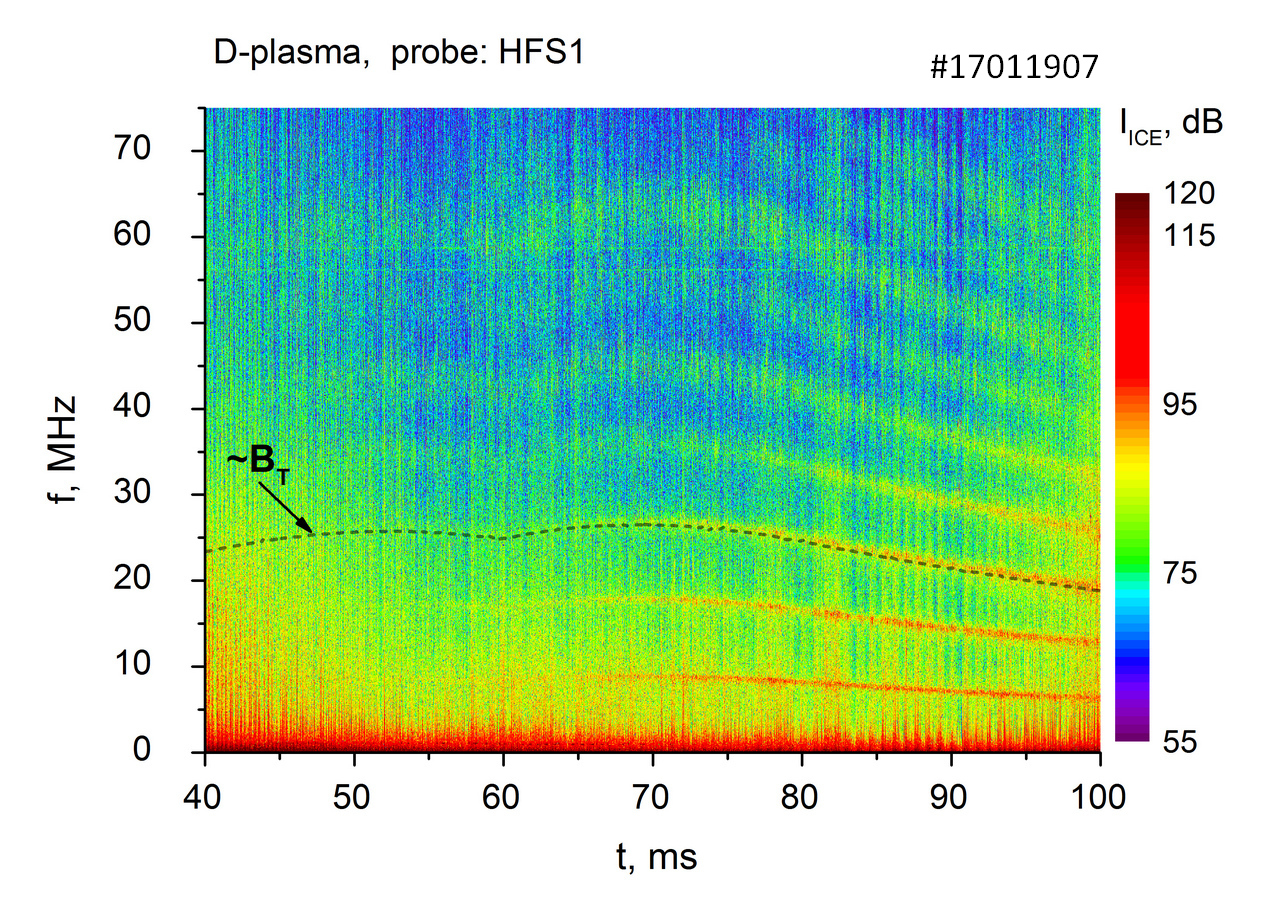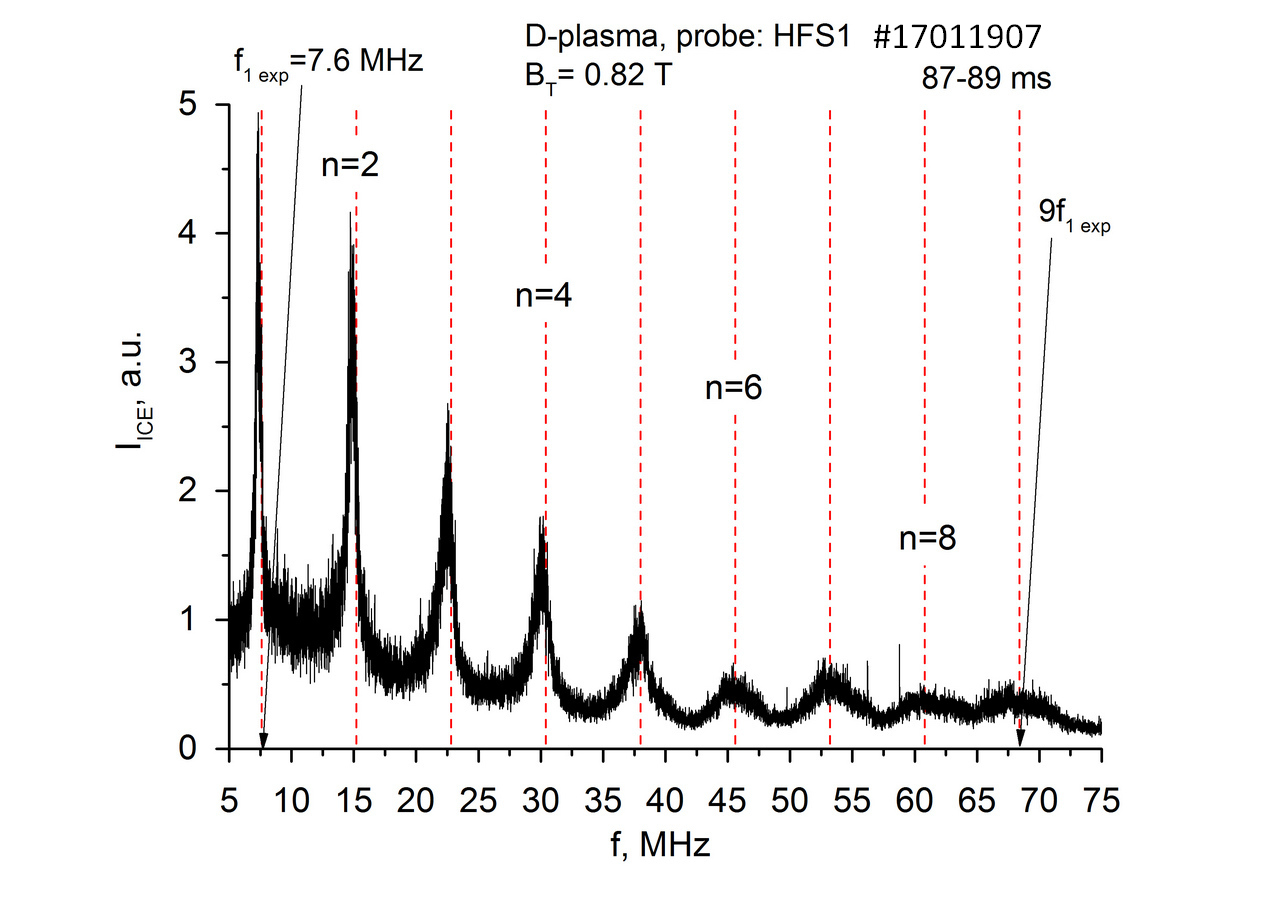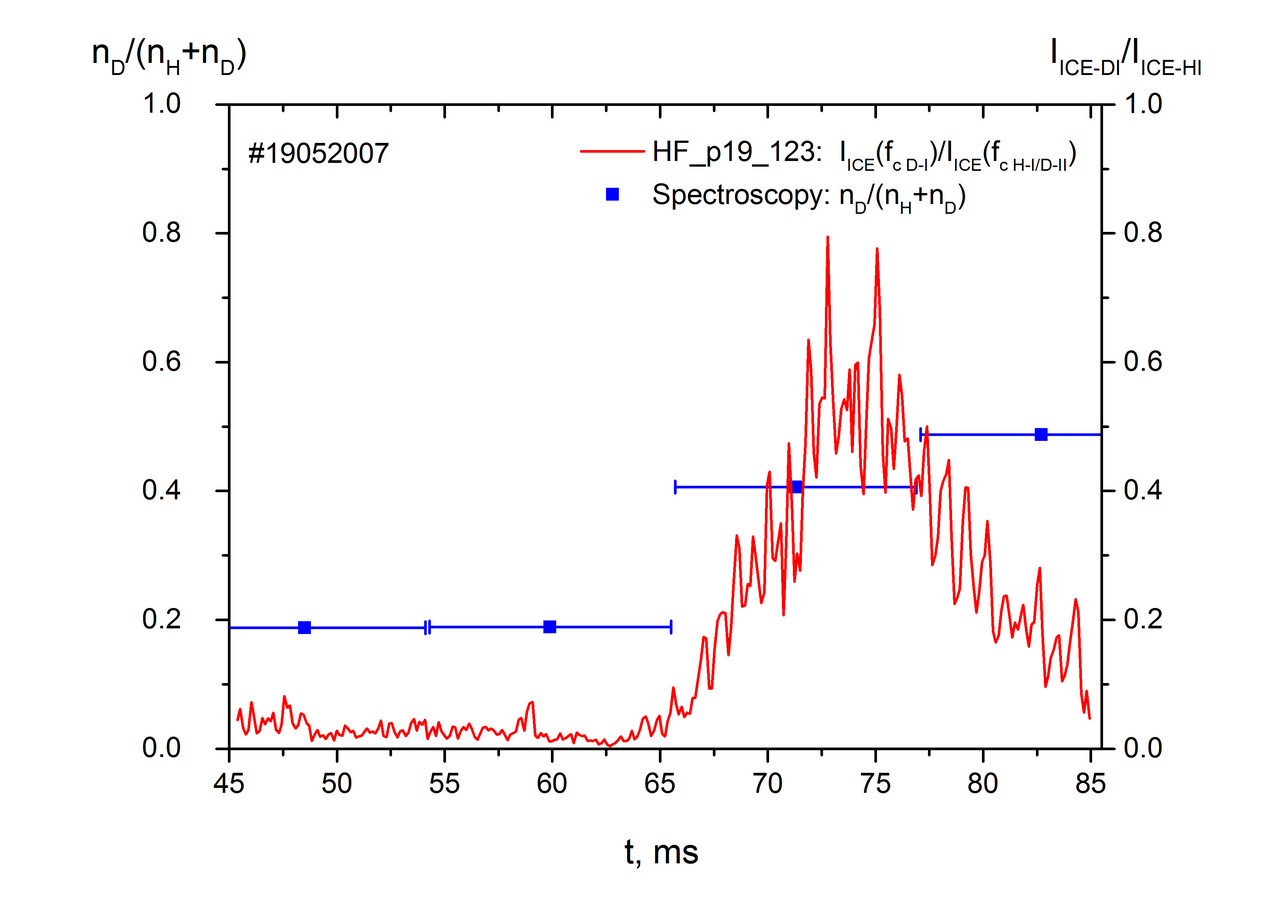Since 18 of December 2019 conferences.iaea.org uses Nucleus credentials. Visit our help pages for information on how to Register and Sign-in using Nucleus.

# 28th IAEA Fusion Energy Conference (FEC 2020)

May 10 – 15, 2021
Virtual Event
Europe/Vienna timezone
The Conference will be held virtually from 10-15 May 2021

## Ion Cyclotron Emission from the ohmically heated plasma in the TUMAN-3M tokamak

May 12, 2021, 2:00 PM
4h 45m
Virtual Event

#### Virtual Event

Regular Poster Magnetic Fusion Experiments

### Speaker

Dr Sergei Lebedev (Ioffe Institute)

### Description

The paper reports unique observation of an ion cyclotron emission (ICE) from the ohmically heated plasma in the absence of energetic ions. The possibility to use the ohmic ICE for determination of the hydrogen isotopes ratio is discussed.

Described experiments were performed in the compact circular shaped limiter tokamak TUMAN-3M $(R(0)=0.53\:m$, $a=0.22\:m$, $B_T=1\:T$, $I_p=150\:kA$, $\overline{n}_e=(1\mbox{-}3)\cdot10^{19}\:m^{-3}$, $Ti(0)=0.2\:keV)$. No auxiliary heating was used in these experiments. The ICE appears as a set of equally spaced lines in the frequency spectra measured by in-vessel magnetic probes. The ICE spectra span from $5$ to $120\:MHz$ depending on a sort of working gas (hydrogen or deuterium) and probe position. In TUMAN-3M an ICE driven by Neutral Beam Injection was observed as well. Study of the NBI driven ICE is presented at this conference in a separate report by Askinazi L.G. et al.

Typical example of the ohmic ICE spectrogram obtained in deuterium plasma (shot #$17011907$) using a signal from the magnetic pick-up probe sited at the high field side of the torus, $R_{probe}=0.43\:m$, is shown on figure 1. The spectrogram contains up to nine harmonics of ICE with the fundamental one matched to the deuteron cyclotron frequency $f_{cD\mbox{-}I}$ at the probe location. Exact proportionality of a harmonic frequency to the local toroidal magnetic field is justified by coincidence of scaled $B_T$ trace with the third harmonic evolution as seen on the figure. The $\sim\;10$% variation of $B_T$, see figure 1, is caused by the features of the TF power supply operation. No variation of the ICE harmonic frequency with density was observed$^1$.ICE spectrum obtained by FFT during $87\mbox{-}89\:ms$ of the shot #$17011907$ is shown on figure 2. The first peak of the spectrum coincides with the deuteron resonance frequency $f_{cD\mbox{-}I}=\omega_{cD\mbox{-}I}/2\pi=7.6\:MHz$ at $R_{probe}=0.43\:m$. Fundamental frequencies of the ICE detected by probes located at other major radii positions strictly match to the cyclotron resonances at the probes’ locations$^1$. The spectrum on figure 2 is characterized by a narrow width of the $f_{cD\mbox{-}I}$ peak. The FWHM of the peak $f_{cD\mbox{-}I}$ is $0.5\:MHz$. The narrowness of the peak indicates that source of the emission is strongly localized in both the major and minor radius directions. The size of the emitting area was estimated to be less than $2.5\:cm$. The peak width increases with harmonic number. FWHM of the fifth harmonic $f_{cD\mbox{-}V}$ is $3\:MHz$. It should be noticed that narrow $f_{cD\mbox{-}I}$ peak is the characteristic of the low density plasma, e.g. in shot #$17011907$ the average plasma density was $1.5\cdot10^{19}\:m^{-3}$. With the increasing density the width of the peak and amplitude of the higher harmonics rise. At average density of $2.7\cdot10^{19}\:m^{-3}$ FWHM of the $f_{cD\mbox{-}I}$ peak attains $2\:MHz$ and $f_{cD\mbox{-}IV}$ or even $f_{cD\mbox{-}V}$ has maximal amplitude in the spectrum.Similar features of the ohmic ICE were found in the shots with hydrogen working gas although amount of observed harmonics was less than in deuterium – 7 instead of 9. The frequency of the fundamental harmonic $f_{cH\mbox{-}I}$ measured by the particular probe was two times higher than the one in deuterium plasma.

Above observations lead to idea to explore a possibility to measure an isotope ratio in the proton/deuteron plasma using the ohmic ICE. In order to do this a set of experiments was performed with additional puffing of deuterium into hydrogen discharge. In addition to the ICE measurements the Alfven oscillations$^2$, optical spectroscopy and neutral particle analysis were used to monitor isotope ratio. Figure 3 presents results of the measurements of relative concentration of deuterium in hydrogen/deuterium plasma – $n_D/(n_H+n_D)$ using optical spectroscopy and ratio of ICE peak intensities at $f_{cD\mbox{-}I}$ and at $f_{cH\mbox{-}I}$ coinciding with $f_{cD\mbox{-}II}$. Data on the figure 3 showed increase in the later from zero to $0.5$ during D-puffing phase. At the same time $n_D/(n_H+n_D)$ reveals similar tendency. These results prove a sensitivity of ICE to the hydrogen isotope ratio. Some disagreement between the ICE and spectroscopy techniques in the pre-puffing phase appears due to the insufficient contrast of the deuterium registration channel for hydrogen scattered light.

The measurement of the isotope ratio is based on the assumption of the linear dependence of intensity of the ICE at fundamental harmonic on the density of emitting ions. In order to be sure in the validity of the assumption the model describing a mechanism of the ohmic ICE generation is necessary. Until now in laboratory experiments ICE was found to be driven by resonant interaction with high energy ion population produced by fusion products or injected beam ions$^{3,4}$. Non-monotonicity or anisotropy of ion distribution function in velocity or physical space is considered as an energy source for the instability$^5$. Since there are no auxiliary heating or fusion reactions in the considered scenario a desired mechanism could not relay on the features of the energy distribution of fast ions and other explanation should exist for the observed ICE.

Two possibilities are considered as possible mechanisms of the ICE generation in the absence of energetic ions. First, the ICE at the multiple harmonics of IC resonance can be generated as a result of Ion Cyclotron Drift Instability (ICDI) excitation in presence of an plasma inhomogeneity$^6$. According to $^6$ the condition of ICDI excitation in single component plasma is: $\rho_i/\alpha>2(m_e/m_i)^{0.5}$, where $\rho_i$ – ion Larmor radius, $\alpha=n/(dn/dr)$ – radial scale of the density gradient, $m_e$ and $m_i$ – electron and ion masses, respectively. Above criterion could be fulfilled in the experimental conditions of TUMAN-3M. In this case relationship between amplitudes of isotope fundamental harmonic depends on ICDI saturation level which will be further analyzed. Another possible mechanism of the ohmic ICE is a thermal emission of gyrating ions. In this case amplitude of fundamental harmonic linearly depends on concentration of the thermal ions and proportional to $\omega^2$ in low to medium dense plasma.

Presented study demonstrates sensitivity of the ICE driven by thermal ions to hydrogen isotope ratio and opens a way to develop novel diagnostic tools for measurement of the isotope ratio in contemporary and future devices.Acknowledgement. The study was supported by RSF grant 16-12-10285 – development of diagnostic tools for ohmic ICE measurements and by Ioffe Institute – support of the tokamak infrastructure.

References
1 Lebedev S.V. et al, EPJ Web Conf., v.149(2017), Art.No #03010
2 Abdullina G.I. et al, Tech. Phys. Lett., v.45(2019), 8, 790
3 Bhadra D.K. et al, Nucl. Fusion, V.26(1986), p.201
4 Cottrell G.A. et al, Nucl. Fusion, V.33(1993), p.1365
5 Coppi B. et al, Phys. Fluids, V.29(1986), p.4060
6 Mikhailovsky A.B., Nucl. Fusion, V.11(1971), p.323

Country or International Organization Russia Ioffe Institute

### Primary authors

Dr Sergei Lebedev (Ioffe Institute) Mrs Gulnara Abdullina (Ioffe Institute) Dr Leonid Askinazi (Ioffe Institute) Mr Alexander Belokurov (Ioffe Institute) Dr Fedor Chernyshev (Ioffe Institute) Dr Vladimir Kornev (Ioffe Institute) Dr Andrey Melnik (Ioffe Institute) Mr Alexander Smirnov (Ioffe Institute) Dr Alexander Tukachinsky (Ioffe Institute) Mr Nickolay Zhubr (Ioffe Institute)